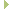Range-v3 Range algorithms, views, and actions for the Standard Libraryranges::v3::distance_fn Struct ReferenceInheritance diagram for ranges::v3::distance_fn:

## Public Member Functions

template<typename Rng , typename D = range_difference_type_t<Rng>, requires = (Integral<D>() && Range<Rng>())>
operator() (Rng &&rng, D d=0) constPublic Member Functions inherited from ranges::v3::iter_distance_fn
template<typename I , typename S , typename D = difference_type_t<I>, requires = (Iterator<I>() && Sentinel<S, I>() && Integral<D>())>
operator() (I begin, S end, D d=0) const